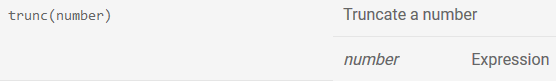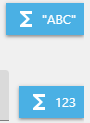# [Solved] Can you trunc a Global Variable?

Can I trunc display a Global Variable?
"Level 1 Time: "+GlobalVariableString(level1time) = shows no decimals, just single figures and works perfectly.

I have tried:

"Level 1 Time: "+ ToString(trunc(GlobalVariable(“level1time”)*100)/100)
"Level 1 Time: "+ ToString(trunc(GlobalVariable(level1time)*100)/100)

Is this possible?
Jim

This is the page you need: Expressions reference [GDevelop wiki]
See the sections Math tools and Text manipulation.Thanks but I cannot find any instructions on how to use the trunc expression:That’s all there is.

As the wiki says, it takes a number as argument.
Don’t forget to convert text/numbers when needed.
Use the blue buttons on the side of the fields to build your line and avoid mistakes.Sorry, tried, it comes up with ToString(trunc(GlobalVariableString(level1time) and works, but there is no mention of using *100/100 or if it can be done.

What’s the point of doing that?level1time is a timer, so if you complete the level in 13:88 seconds, the normal code rounds it to 14 seconds.
I would like it to show as 13:88 so you want to beat it.

“Tempo: “+ToString(round((TimerElapsedTime(“tempo”)*10)/10))+” s” result is a integer

“Tempo: “+ToString(floor(TimerElapsedTime(“tempo”)*10)/10)+” s” result is a float

Thanks, but that does not work either.
Jim

If you want “:” as separator, you’ll have to use Text manipulation expressions, I think.
Take the first two characters, add the sign, and add the next two characters.

Done it.
So…when I hit the goal, I was setting the GV to be single/normal with this:
round(TimerElapsedTime(“level1timer”))

Then I was trying to show/write the GV on another scene using ToString(trunc(GlobalVariable(“level1time”)*100)/100)

but what I needed to do was set the goal GV to this:
trunc(TimerElapsedTime(“level1timer”)*100)/100

then display on the other scene with this:
GlobalVariableString(level1time)

Thanks, game out in 2 days!
Jim

1 Like